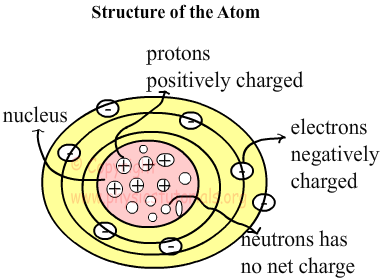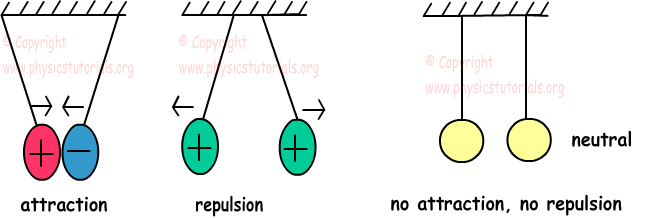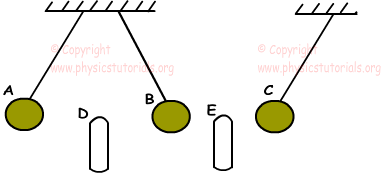ELECTROSTATICS

Scientist found that if you rub an ebonite rod into silk you observe that rod pulls the paper pieces. Or in winter when you put off your pullover, your hair will be charged and move. We first examine the structure of atom to understand electricity better. Experiments done show that there are three types of particle in the atom. Two of them are placed at the center (nucleus) of the atom which we called proton (p) and neutron (n). Proton has positive charges “+” and neutron has no net charge. Third particle is called electron (e) and they are placed at the orbits of the atom. They are negatively charged “-”. Electrons can move but proton and neutron of the atom are stationary.

We show charge with “q” or “Q” and smallest unit charge is 1.6021x10-19 Coulomb (C). One electron and a proton have same amount of charge.

Positively Charged Particles

In this type of particles, numbers of positive ions are larger than the numbers of negative ions. In other words numbers of protons are larger than the number of electrons.

p+>e-

To neutralize positively charged particles, electrons from the surroundings come to this particle until the number of protons and electrons become equal. Do not forget protons cannot move!

Negatively Charged Particles

In this type of particles, numbers of negative ions are larger than the numbers of positive ions. In other words numbers of electrons are larger than the number of protons.

e+>p-

To neutralize negatively charged particles, since protons cannot move and cannot come to negatively charged particles, electrons moves to the ground or any other particle around itself.

Neutral Particles

These types of particles include equal numbers of protons and electrons. Be careful, they have both protons, neutrons and electrons however, numbers of “+” ions are equal to the numbers of “-” ions.

e+=p-

Conductors

Some of the matters have lots of free electrons to move. It is easy for electrons to flow from these materials. Metals are good conductors. Gold, copper, human bodies, acid, base and salt solutions are example of conductors.

Insulators

These types of materials do not let electrons flow. Bonds of the electrons in the insulators are tighter than the conductors thus, they cannot move easily. Glass, ebonite, plastic, wood, air are some of the examples of insulators.

Atoms having same charge repel each other and atoms having opposite charges attract each other.Example: Charged spheres A, B and C behave like this under the effect of charged rod D and E. If C is positively charged, find the signs of the other spheres and rods.We learned that opposite charges attract each other and same charges repel each other. Using this explanation we can say that, if the sign of the C is “+” than rod E must be “-” since it attracts C. B must be “+” since E also attract B. Rod D repels the B so, we say that D must have same sign with B “+” , and finally D also repels A, thus A is also “+”.

A(+), D(+), B(+), E(-), C(+)

Electrostatics Exams and Solutions

Related